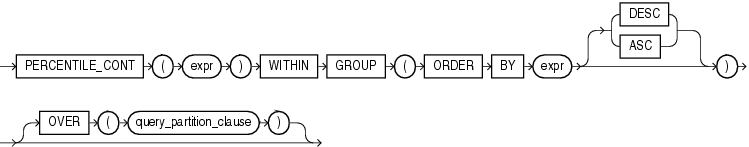## PERCENTILE_CONT

SyntaxDescription of the illustration percentile_cont.eps

for information on syntax, semantics, and restrictions of the `OVER` clause

Purpose

`PERCENTILE_CONT` is an inverse distribution function that assumes a continuous distribution model. It takes a percentile value and a sort specification, and returns an interpolated value that would fall into that percentile value with respect to the sort specification. Nulls are ignored in the calculation.

This function takes as an argument any numeric data type or any nonnumeric data type that can be implicitly converted to a numeric data type. The function returns the same data type as the numeric data type of the argument.

Table 2-8 for more information on implicit conversion

The first `expr` must evaluate to a numeric value between 0 and 1, because it is a percentile value. This `expr` must be constant within each aggregation group. The `ORDER` `BY` clause takes a single expression that must be a numeric or datetime value, as these are the types over which Oracle can perform interpolation.

The result of `PERCENTILE_CONT` is computed by linear interpolation between values after ordering them. Using the percentile value (P) and the number of rows (N) in the aggregation group, you can compute the row number you are interested in after ordering the rows with respect to the sort specification. This row number (RN) is computed according to the formula `RN` `=` `(1+(P*(N-1))`. The final result of the aggregate function is computed by linear interpolation between the values from rows at row numbers `CRN` `=` `CEILING(RN)` and `FRN` `=` `FLOOR(RN)`.

The final result will be:

```  If (CRN = FRN = RN) then the result is
(value of expression from row at RN)
Otherwise the result is
(CRN - RN) * (value of expression for row at FRN) +
(RN - FRN) * (value of expression for row at CRN)
```

You can use the `PERCENTILE_CONT` function as an analytic function. You can specify only the `query_partitioning_clause` in its `OVER` clause. It returns, for each row, the value that would fall into the specified percentile among a set of values within each partition.

The `MEDIAN` function is a specific case of `PERCENTILE_CONT` where the percentile value defaults to 0.5. For more information, refer to MEDIAN.

Note:

Before processing a large amount of data with the `PERCENTILE_CONT` function, consider using one of the following methods to obtain approximate results more quickly than exact results:

• Set the `APPROX_FOR_PERCENTILE` initialization parameter to `PERCENTILE_CONT` or `ALL` before using the `PERCENTILE_CONT` function. Refer to Oracle Database Reference for more information on this parameter.

• Use the `APPROX_PERCENTILE` function instead of the `PERCENTILE_CONT` function. Refer to APPROX_PERCENTILE.

Aggregate Example

The following example computes the median salary in each department:

```SELECT department_id,
PERCENTILE_CONT(0.5) WITHIN GROUP (ORDER BY salary DESC) "Median cont",
PERCENTILE_DISC(0.5) WITHIN GROUP (ORDER BY salary DESC) "Median disc"
FROM employees
GROUP BY department_id
ORDER BY department_id;

DEPARTMENT_ID Median cont Median disc
------------- ----------- -----------
10        4400        4400
20        9500       13000
30        2850        2900
40        6500        6500
50        3100        3100
60        4800        4800
70       10000       10000
80        8900        9000
90       17000       17000
100        8000        8200
110       10154       12008
7000        7000
```

`PERCENTILE_CONT` and `PERCENTILE_DISC` may return different results. `PERCENTILE_CONT` returns a computed result after doing linear interpolation. `PERCENTILE_DISC` simply returns a value from the set of values that are aggregated over. When the percentile value is 0.5, as in this example, `PERCENTILE_CONT` returns the average of the two middle values for groups with even number of elements, whereas `PERCENTILE_DISC` returns the value of the first one among the two middle values. For aggregate groups with an odd number of elements, both functions return the value of the middle element.

Analytic Example

In the following example, the median for Department 60 is 4800, which has a corresponding percentile (`Percent_Rank`) of 0.5. None of the salaries in Department 30 have a percentile of 0.5, so the median value must be interpolated between 2900 (percentile 0.4) and 2800 (percentile 0.6), which evaluates to 2850.

```SELECT last_name, salary, department_id,
PERCENTILE_CONT(0.5) WITHIN GROUP (ORDER BY salary DESC)
OVER (PARTITION BY department_id) "Percentile_Cont",
PERCENT_RANK()
OVER (PARTITION BY department_id ORDER BY salary DESC) "Percent_Rank"
FROM employees
WHERE department_id IN (30, 60)
ORDER BY last_name, salary, department_id;

LAST_NAME                     SALARY DEPARTMENT_ID Percentile_Cont Percent_Rank
------------------------- ---------- ------------- --------------- ------------
Austin                          4800            60            4800           .5
Baida                           2900            30            2850           .4
Colmenares                      2500            30            2850            1
Ernst                           6000            60            4800          .25
Himuro                          2600            30            2850           .8
Hunold                          9000            60            4800            0
Khoo                            3100            30            2850           .2
Lorentz                         4200            60            4800            1
Pataballa                       4800            60            4800           .5
Raphaely                       11000            30            2850            0
Tobias                          2800            30            2850           .6```